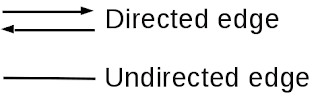## Introduction to graph theory

In mathematics and computer science, graph theory is the study of graphs.  A graph represents relationship between objects. I am going to use this theory to represent relationship between authors. It is straightforward to use graph theory to understand the relationships between objects. In that sense, this is nothing new, but I am curious about the application of this method to understand an aspect of literature --- the relationship between authors.

## Fundamental objects of graph

Graph theory has two fundamental objects: a node (also called a vertex) and an edge. A graph, then, is a set of nodes connected by edges. If two nodes are connected by an edge, these two nodes are defined to have a relationship.

Figure 1 shows these fundamental objects. Graph theory only thinks about these two objects. You might think that this is simple, since there are only two types of objects. However, we will see that complexity is still possible.Figure 1. Node and Edge.

Usually, there is more than one node in a graph. To distinguish multiple nodes, we typically put numbers on them (as shown in Figure 2).  However, since the purpose of using number is to distinguish the nodes, we don't have to use numbers. However, numbering nodes is the easiest way to distinguish them, so many of the examples will use numbers.Figure 2. To distinguish different nodes, put the numbers on them.

There are two types of edges shown in Figure 3: a directed edge and an undirected edge. The difference between them is that one has a direction and the other has no direction, which usually means it represents both directions. These types reflect the two type of relations: a one-directional relation and a bi-directional relation. For example, a person in the past can effect a person today with their writing but someone today cannot afect someone in the past.  This relation is one-directional.Figure 3. Directed and undirected edge.

Today, I show you who are the dramatis personae in graph theory. They are node and edge. So far, so simple. Next time I would like to talk about what is graph.